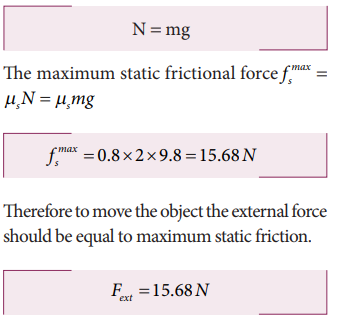Home | | Physics 11th std | Static Friction

# Static Friction

Static friction is the force which opposes the initiation of motion of an object on the surface.

Static Friction (vec fs)

Static friction is the force which opposes the initiation of motion of an object on the surface. When the object is at rest on the surface, only two forces act on it. They are the downward gravitational force and upward normal force. The resultant of these two forces on the object is zero. As a result the object is at rest as shown in Figure 3.23.If some external force Fext is applied on the object parallel to the surface on which the object is at rest, the surface exerts exactly an equal and opposite force on the object to resist its motion and tries to keep the object at rest. It implies that external force and frictional force are exactly equal and opposite. Therefore, no motion parallel to the surface takes place. But if the external force is increased, after a particular limit, the surface cannot provide sufficient opposing frictional force to balance the external force on the object. Then the object starts to slide. This is the maximal static friction that can be exerted by the surface. Experimentally, it is found that the magnitude of static frictional force fs satisfies the following empirical relation.where µs is the coefficient of static friction. It depends on the nature of the surfaces in contact. N is normal force exerted by the surface on the body and sometimes it is equal to mg. But it need not be equal to mg always.

Equation (3.27) implies that the force of static friction can take any value from zero to µsN.

If the object is at rest and no external force is applied on the object, the static friction acting on the object is zero ( fs = 0).

If the object is at rest, and there is an external force applied parallel to the surface, then the force of static friction acting on the object is exactly equal to the external force applied on the object ( f s = Fext ). But still the static friction fs is less than µsN.

When object begins to slide, the static friction ( fs) acting on the object attains maximum,

The static and kinetic frictions (which we discuss later) depend on the normal force acting on the object. If the object is pressed hard on the surface then the normal force acting on the object will increase. As a consequence it is more difficult to move the object. This is shown in Figure 3.23 (a) and (b). The static friction does not depend upon the area of contact.

The values of coefficient of static friction for pairs of materials are presented in Table 3.1. Note that the ice and ice pair have very low coefficient of static friction. This means a block of ice can move easily over another block of ice.## Solved Example Problems for Static Friction

### Example 3.17

Consider an object of mass 2 kg resting on the floor. The coefficient of static friction between the object and the floor is µ s = 0.8. What force must be applied on the object to move it?

### Solution

Since the object is at rest, the gravitational force experienced by an object is balanced by normal force exerted by floor.Therefore to move the object the external force should be equal to maximum static friction.

### Example 3.18

Consider an object of mass 50 kg at rest on the floor. A Force of 5 N is applied on the object but it does not move. What is the frictional force that acts on the object?

### Solution

When the object is at rest, the external force and the static frictional force are equal and opposite.

The magnitudes of these two forces are equal, f s = Fext

Therefore, the static frictional force acting on the object is

Fs=5N

The direction of this frictional force is opposite to the direction of Fext .

### Example 3.19

Two bodies of masses 7 kg and 5 kg are connected by a light string passing over a smooth pulley at the edge of the table as shown in the figure. The coefficient of static friction between the surfaces (body and table) is 0.9. Will the mass m1 = 7 kg on the surface move? If not what value of m2 should be used so that mass 7 kg begins to slide on the table?

### Solution

As shown in the figure, there are four forces acting on the mass m1

a) Downward gravitational force along the negative y-axis (m1g)

b) Upward normal force along the positive y axis (N)

c) Tension force due to mass m2 along the positive x axis

d) Frictional force along the negative x axis

Since the mass m2 has no vertical motion, m1= NTo determine whether the mass m1 moves on the surface, calculate the maximum static friction exerted by the table on the mass m1. If the tension on the mass m1 is equal to or greater than this maximum static friction, the object will move.The tension T = m2 g = 5X9.8 = 49 NThe tension acting on the mass m1 is less than the maximum static friction. So the mass m1 will not move.

To move the mass m1T = fsmax where T = m2gIf the mass m2 is 6.3 kg then the mass m1 will begin to slide. Note that if there is no friction on the surface, the mass m1 will move for m2even for just 1 kg.

The values of coefficient of static friction for pairs of materials are presented in Table 3.1. Note that the ice and ice pair have very low coefficient of static friction. This means a block of ice can move easily over another block of ice.

Tags : with Solved Example Problems , 11th Physics : UNIT 3 : Laws of Motion
Study Material, Lecturing Notes, Assignment, Reference, Wiki description explanation, brief detail
11th Physics : UNIT 3 : Laws of Motion : Static Friction | with Solved Example Problems